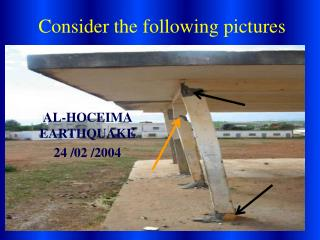DownloadDownload PresentationConsider the following pictures

# Consider the following pictures

Download Presentation## Consider the following pictures

- - - - - - - - - - - - - - - - - - - - - - - - - - - E N D - - - - - - - - - - - - - - - - - - - - - - - - - - -
##### Presentation Transcript

1. Consider the following pictures AL-HOCEIMA EARTHQUAKE 24 /02 /2004

2. Consider the following pictures

3. Consider the following pictures

4. Robert Hooke 1635-1703 • Researched and wrote papers on Geometry, Snowflakes, Heat, Astronomy, Fossils, Air Pumps, Light, Watches, Telescopes and Silkworms…..among other things. • Hooke’s Law was one of the few things he was popularly credited with creating. • A rather crabby individual but credited with also inventing the universal joints, the balance wheel and the iris diaphragm

5. What did Hooke discover about forces ? • the more force that was put on materials the more they extended • With some materials they also extended in a regular way e.g.- if the force was doubled so did the extension • this was true as long as their elastic limit was not exceeded

6. Lets try an activity • Read the instructions on lab sheet provided. • Arrange apparatus and perform experiments. • Analyse and present data

7. What did the experiment show?

8. What the graph shows

9. What is the elastic limit? • The material no longer shows elastic behaviour (i.e. does not return to original size when stretching force is removed) • The material is permanently deformed i.e. is larger or longer than originally • The material is weaker as the above effects are caused by fracture of some atomic bonds

10. Hence in general, the Forceis proportional toextension.SoHooke’s Law could be put as Where F is the applied force in Newtons x is the extension in metres Or if k is the proportionality constant F=kx

11. What does k mean in F=kx? • k is called the spring constant and is a measure of the stiffness of the spring or material • It has units of Nm-1 (newtons per metre) • The higher the k the stiffer the spring • Materials with a high k need a large force to for a given extension • adding springs in series or parallel changes k

12. k decreases by 2 Combined springs slacken compared with single spring extension doubles Series and parallel springs Parallel Series k increases by 2 Combined springs stiffen compared with single spring extension halves

13. So how does this help me? • Understanding Hooke’s law is critical to the construction of any structure eg bridges or buildings. • During earthquakes deformations of buildings occur.

14. Summary Hooke’s law explains how a material’s extension/deformation depends on the applied force.Deformation of materials though in practice is looked at in terms of stress ( the force per square metre) and strain (the extension per unit length) are more commonly used instead of simply force and extension